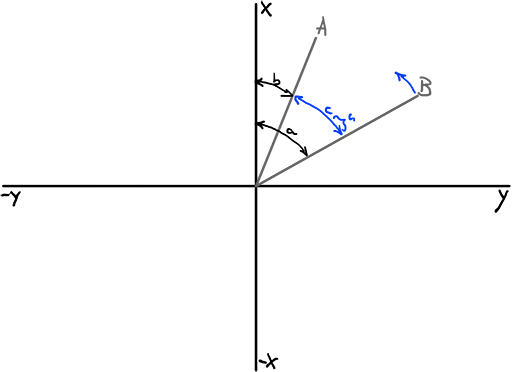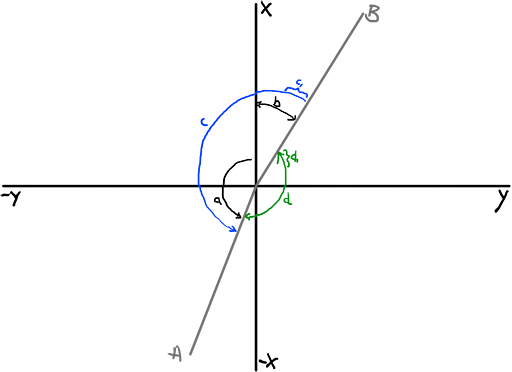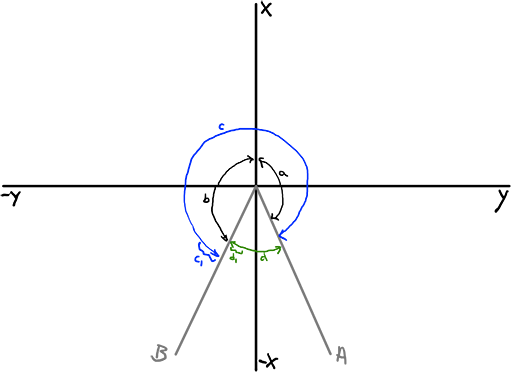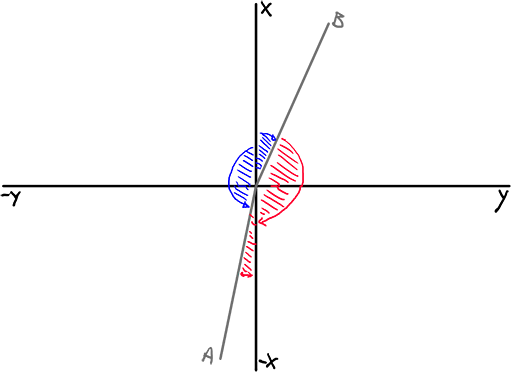### Lerping between two anglesPublished on 9/15/2021

Lerping between two yaw angles is a problem that’s harder to solve than it appears at first. At least, that’s my experience with trying to deal with it. I’ve solved it twice now, for slightly different end-goals.

I’m building a tracking turret. The turret is given a designated target (A). The turret is facing in a different direction (B). It has to orient itself towards the target. Clearly the solution here is to figure out the angle towards the target from the current orientation, and lerp to it the amount that can be moved in the limited time of a single frame update/tick. Not considering that Unreal Engine reports a vector's heading as ranging from -180° to +180° with 0° on the X axis, and ignoring the lerp, we have

c = (A - B)

Now we can calculate c1 as say a 10% lerp to create an ease-in towards the target.For example, the turret is facing 50°, and has to rotate to 20°.

c1 = (20° - 50°) * 10% = -3°

It correctly rotates that small amount in the negative direction. So you build this, but things don’t work so well. There are some scenarios where it just assumes some wild angles. Why? Consider:c1 = ((-150)° - 40°) * 10% = -19°

Notice that the result is negative, which means the turret is going to turn CCW here. This naive calculation only considers the reflex angle c instead of the obtuse angle d. Swop A and B around, and we have

c1 = (40° - (-150°)) * 10% = 19°

This is also the wrong direction. `(-150°) + 19° = -131°` which is rotating towards the reflex angle instead of the obtuse angle d.

So now we have a branch in our algorithm: Both A and B are the same sign, or A and B are separate signs. At this point you might explore the idea of branching on “if there is an acute angle between them or not”. But this isn’t exhaustive enough. Consider:c1 = (150° - (-150°)) * 10% = 30°

There is a 60° acute angle between them, but we’re rotating towards the reflex angle instead of the acute angle. And when A and B are swapped, we have the same problem in the other direction:

c1 = ((-150°) - 150°) * 10% = -30°

So, we have to consider the signs instead. And then, when they do have a separate sign, we have to figure out which subtraction yields you the obtuse angle instead of the reflex angle. Consider:c1 = ((-170°) - 30°) * 10% = -20°

This incorrectly considers the blue reflex angle. Swapping it around (the formula, not the input target/destination) gives us the correct (positive CW) direction, but the wrong amount of degrees:

c1 = (30° - (-170°)) * 10% = 20°

So here we need to check if the absolute resulting difference is a reflex angle (> 180), and take the remaining obtuse angle as positive. We know we need it as positive because the only way to be in this scenario is when the naïve difference is a reflex angle combined with starting in a positive angle and rotating towards a negative angle.

Conversely, if we started in a negative angle, and need to rotate towards a positive angle, and our naïve difference is a reflex angle, the result should always be negative so that the rotation is CCW.

So, this is quite contrived and non-trivial to implement. I have to question if it might be possible to use the dot product between the two vectors to determine some of these edge cases? I'm not sure since I haven't looked into that, but it feels possible. Unreal Engine's "Lerp (Rotator)" function doesn't solve this problem, even with the "Shortest Path" bool ticked. And I don't know the Unity3D math library that well. If you know of a way to solve this more easily or straight forward using an advanced math function/assumption/statement that I have forgotten about, please drop me a comment or DM.